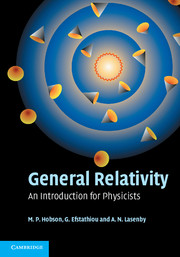Home > Catalog > General RelativitySearch this book## Details

• 94 b/w illus. 8 tables 368 exercises
• Page extent: 592 pages
• Size: 247 x 174 mm
• Weight: 1.321 kg

## Library of Congress

• Dewey number: 530.11
• Dewey version: 22
• LC Classification: QC173.6 .H63 2006
• LC Subject headings:
• General relativity (Physics)

Library of Congress Record

### Hardback

(ISBN-13: 9780521829519 | ISBN-10: 0521829518)

#### Manufactured on demand: supplied direct from the printer

##### \$94.99 (X)

After reviewing the basic concept of general relativity, this introduction discusses its mathematical background, including the necessary tools of tensor calculus and differential geometry. These tools are used to develop the topic of special relativity and to discuss electromagnetism in Minkowski spacetime. Gravitation as spacetime curvature is introduced and the field equations of general relativity derived. After applying the theory to a wide range of physical situations, the book concludes with a brief discussion of classical field theory and the derivation of general relativity from a variational principle.

### Contents

1. The spacetime of special relativity; 2. Manifolds and coordinates; 3. Vector calculus on manifolds; 4. Tensor calculus on manifolds; 5. Special relativity revisited; 6. Electromagnetism; 7. The equivalence principle and spacetime curvature; 8. The gravitational field equations; 9. The Schwarzschild geometry; 10. Experimental tests of general relativity; 11. Schwarzschild black holes; 12. Further spherically-symmetric geometries; 13. The Kerr geometry; 14. The Friedmann–Robertson–Walker geometry; 15. Cosmological models; 16. Inflationary cosmology; 17. Linearised general relativity; 18. Gravitational waves; 19. A variational approach to general relativity.

### Reviews

"General Relativity is written clearly... gives a good, readable introduction to the foundations and applications of general relativity theory, and it is a good choice for a general relativity course emphasizing astrophysical and cosmological applications."
Lawrence H. Ford, Tufts University for Physics Today

"Like any good book on general relativity, much is expected of the reader, but the writing is concise and elegant, with plenty of good exercises for the student to work on. The authors strike an excellent balance between the demands of mathematical rigor and physical significance."
Alan S.McRae, Mathematical Reviews